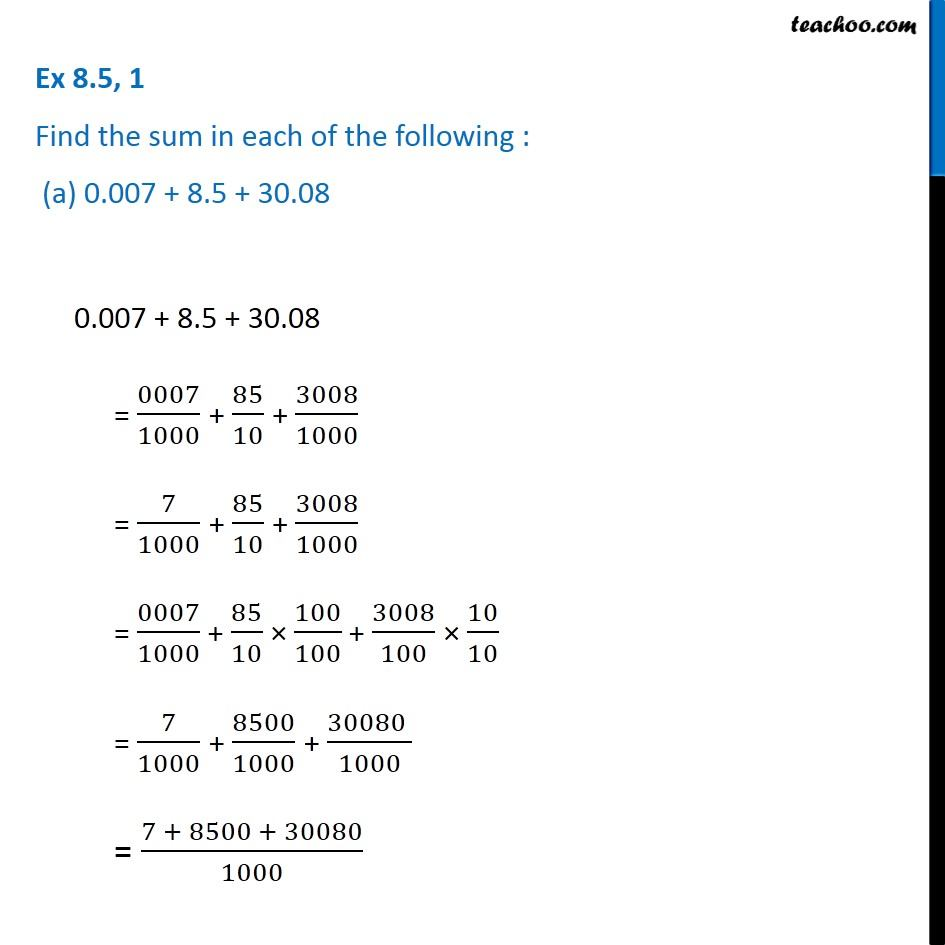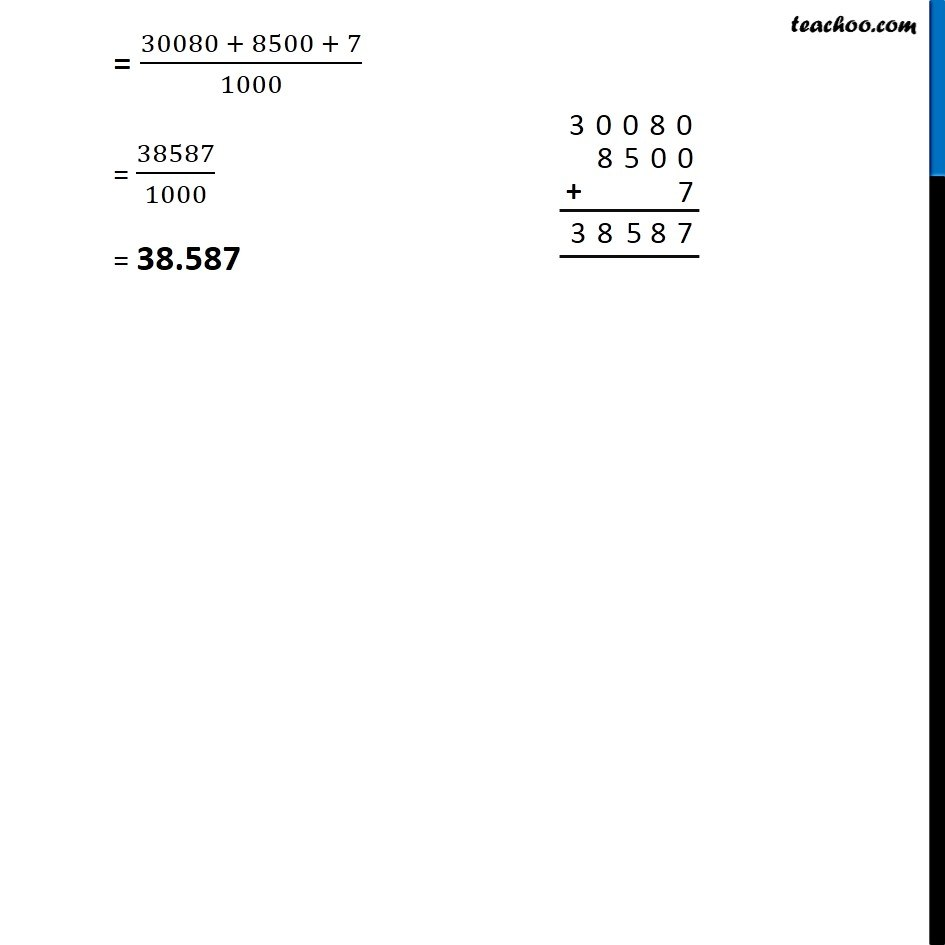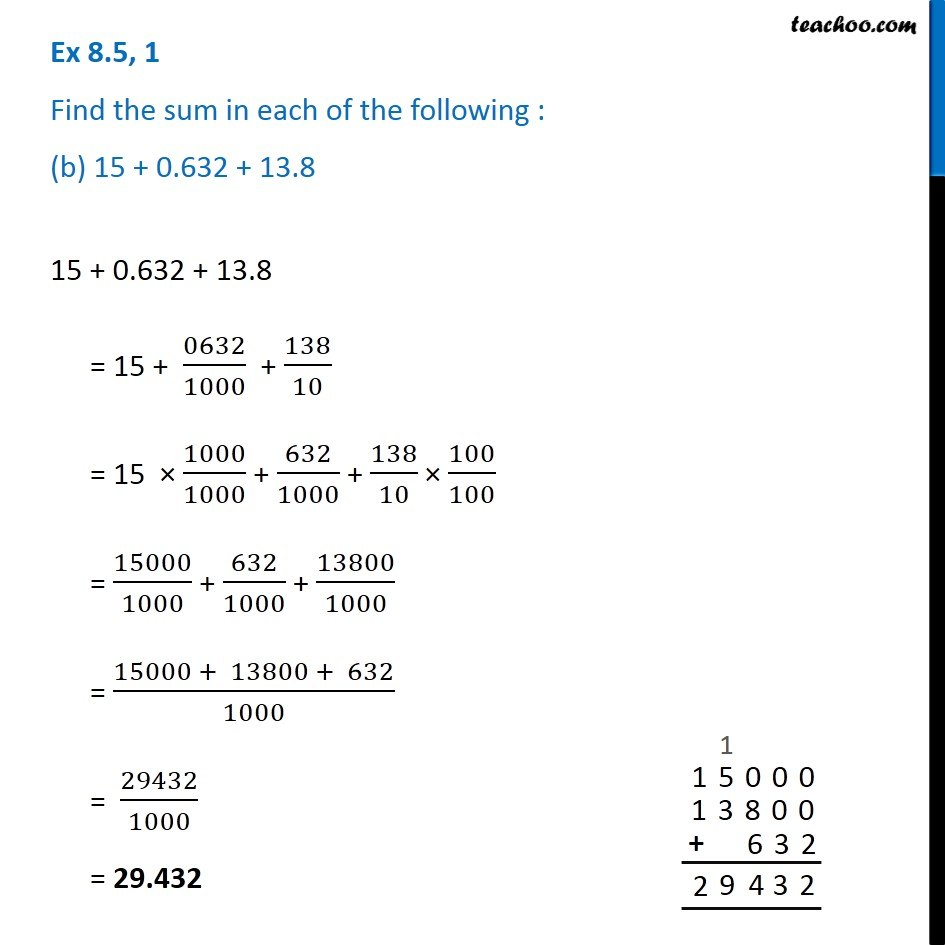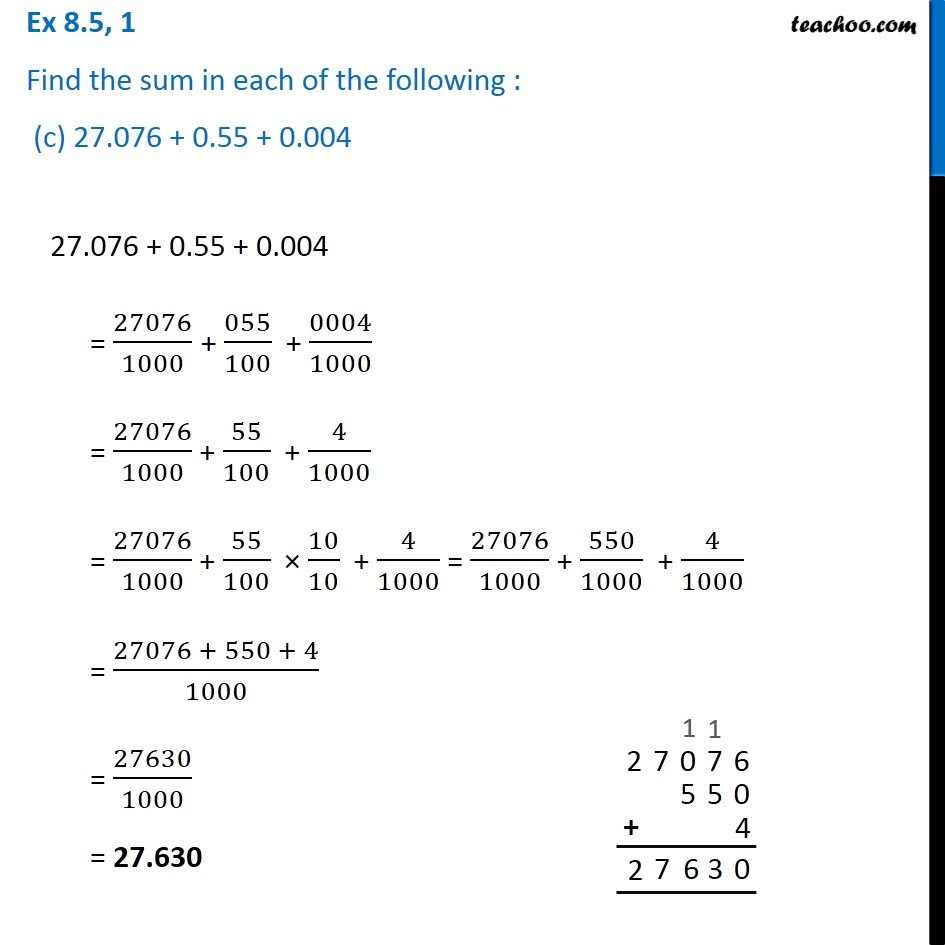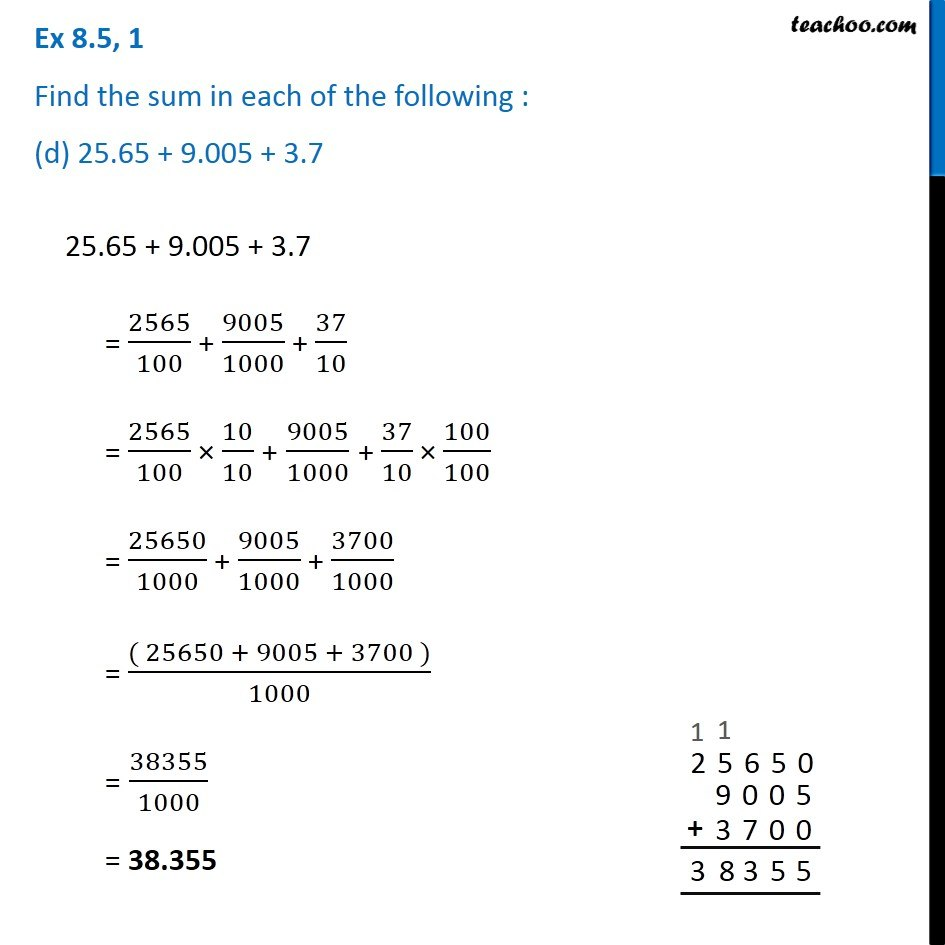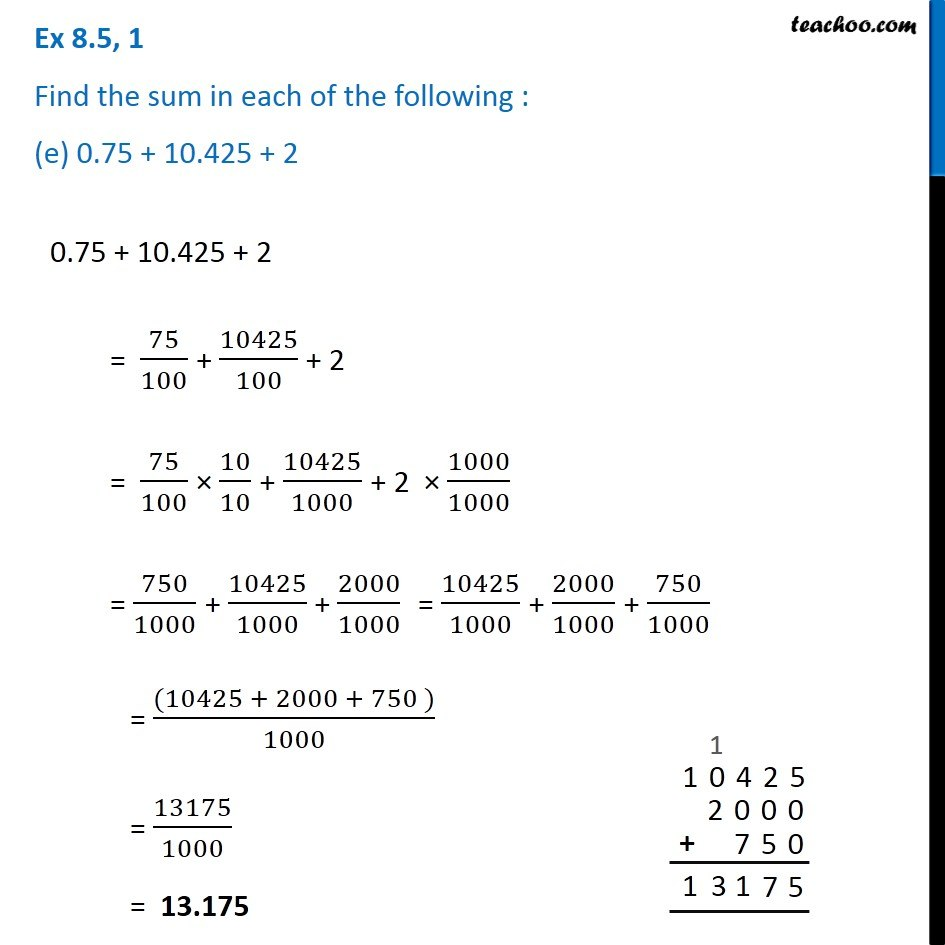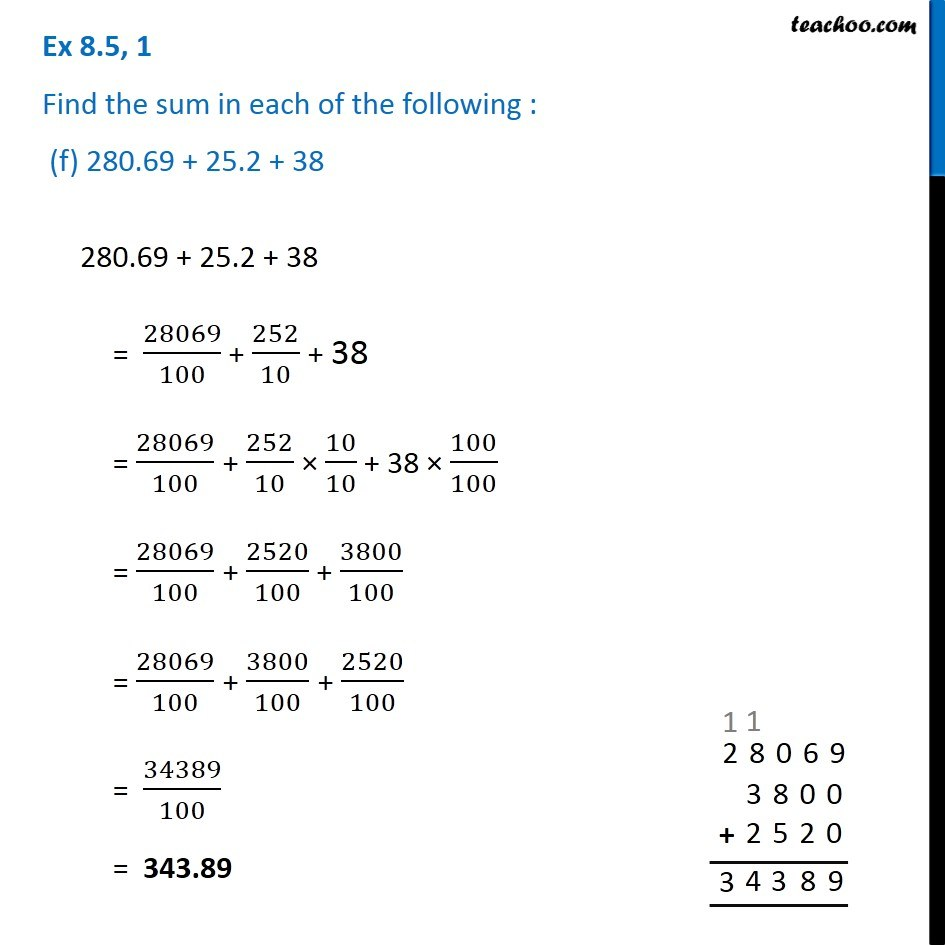Subscribe to our Youtube Channel - https://you.tube/teachoo

1. Chapter 8 Class 6 Decimals
2. Serial order wise
3. Ex 8.5

Transcript

Ex 8.5, 1 Find the sum in each of the following : (a) 0.007 + 8.5 + 30.080.007 + 8.5 + 30.08 = 0007/1000 + 85/10 + 3008/1000 = 7/1000 + 85/10 + 3008/1000 = 0007/1000 + 85/10 × 100/100 + 3008/100 × 10/10 = 7/1000 + 8500/1000 + (30080 )/1000 = (7 + 8500 + 30080)/1000 = (30080 + 8500 + 7)/1000 = 38587/1000 = 38.587 Ex 8.5, 1 Find the sum in each of the following : (b) 15 + 0.632 + 13.815 + 0.632 + 13.8 = 15 + 0632/1000 + 138/10 = 15 × 1000/1000 + 632/1000 + 138/10 × 100/100 = 15000/1000 + 632/1000 + 13800/1000 = (15000 + 13800 + 632)/1000 = 29432/1000 = 29.432 Ex 8.5, 1 Find the sum in each of the following : (c) 27.076 + 0.55 + 0.00427.076 + 0.55 + 0.004 = 27076/1000 + 055/100 + 0004/1000 = 27076/1000 + 55/100 + 4/1000 = 27076/1000 + 55/100 × 10/10 + 4/1000 = 27076/1000 + 550/1000 + 4/1000 = (27076 + 550 + 4)/1000 = 27630/1000 = 27.630 Ex 8.5, 1 Find the sum in each of the following : (d) 25.65 + 9.005 + 3.725.65 + 9.005 + 3.7 = 2565/100 + 9005/1000 + 37/10 = 2565/100 × 10/10 + 9005/1000 + 37/10 × 100/100 = 25650/1000 + 9005/1000 + 3700/1000 = (( 25650 + 9005 + 3700 ))/1000 = 38355/1000 = 38.355 Ex 8.5, 1 Find the sum in each of the following : (e) 0.75 + 10.425 + 20.75 + 10.425 + 2 = 75/100 + 10425/100 + 2 = 75/100 × 10/10 + 10425/1000 + 2 × 1000/1000 = 750/1000 + 10425/1000 + 2000/1000 = 10425/1000 + 2000/1000 + 750/1000 = ((10425 + 2000 + 750 ))/1000 = 13175/1000 = 13.175 Ex 8.5, 1 Find the sum in each of the following : (f) 280.69 + 25.2 + 38 280.69 + 25.2 + 38 = 28069/100 + 252/10 + 38 = 28069/100 + 252/10 × 10/10 + 38 × 100/100 = 28069/100 + 2520/100 + 3800/100 = 28069/100 + 3800/100 + 2520/100 = 34389/100 = 343.89

Ex 8.5

Chapter 8 Class 6 Decimals
Serial order wise